# Spring 2022 Final Exam Solution for Statistical Analysis in R North Carolina State University

Below are the questions and answers to the data analysis final exam done in the Spring of 2022. You can use these solutions as a guide on how to complete your statistical analysis in R test questions. If you ever need a professional to take your statistics exams, you can always contact us with the exam instructions and we will help you out.

## Exam Question: Write a business case to identify problems related to dataset

Write a business case where you identify a problem related to a dataset and consider solutions by use of statistical analysis. Create an introduction, a description of the company and its location, and the different ratings of the chocolate bars.

Exam Solution:

### Introduction: Analysis of Chocolate Bars

The dataset contains the details of expert reviews about 1700 chocolate bars, which are consumed in the US. The data points about each chocolate bar consist of the Company, Bean Origin, Year of Review by the expert, Cocoa Percentage in the chocolate bar, Company Location, Rating given by the expert, type of bean, and the broad bean origin.

### Company Location

The companies are located in 59 countries with the US being the most common country for the companies. The following image shows the frequency table of the companies in the dataset.There are 764 chocolate bars which are manufactured by companies in the US, followed by 156 firms in France, 125 in Canada, and 96 in the UK. There are 38 types of chocolate bars manufactured in Switzerland and 40 in Belgium.

### Ratings of chocolate bars

The chocolate bars have been rated on a scale from 1 to 5, with rating increments of 0.25. The ratings assigned are approximately symmetric and resemble a left-skewed distribution.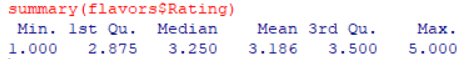The average rating assigned is 3.186, with the median close by at 3.25. The 25th percentile of ratings is 2.875 and the 75th percentile is 3.5. The maximum ratings assigned are 5 and the minimum is 1

The following table shows the frequency distribution of the ratings.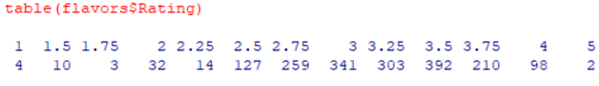As indicated by the summary statistics, most of the ratings are clustered between 2.5 and 3.75, with very few ratings beyond either side of the range. There are 4 chocolate bars that have been rated as 1 and 2 chocolate bars were rated 5.

The following figure shows the histogram of the ratings assigned to the chocolate bars.The histogram appears to be skewed leftwards, due to the presence of more outliers on the left side of the scale.

## Exam Question: Where are the most favored cacao beans grown?

Exam Solution

### Origin of most favored cocoa beans

The variable Borad.Bean.Origin can be used as an indicator of the bean origin and the ratings assigned by the experts can be the measure of the degree of favoredness of the chocolate bars.

There are 100 locations from the cocoa beans originated. We will use the tapply() from the dplyr package to measure the average ratings assigned to each origin/location of the cocoa bean and use it to figure out the top 5 most favored origins of the cocoa beans.

The following picture shows the origins of the most favored beans, with their average ratings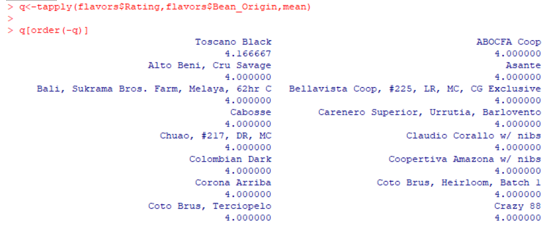Toscano Black has the highest average rating of all the bean origins, at 4.1667, followed by a number of locations such as ABOCFA Coop, Asante, etc, which have been rated 4 by the experts.

## Exam Question: Which companies have the highest-rated chocolate bars?

Exam Solution

The variable Company records the company name of the manufacturer of the chocolate bars. We can use apply () function to figure out the list of firms with the highest-rated chocolate bars.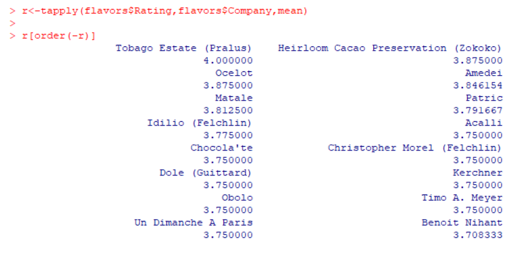The company Tobago Estate (Pralus) produces the highest-rated chocolate bars, with an average rating of 4.00, followed by Heirloom Cacao Preservation (Zokoko) and Ocelot with an average rating of 3.875.

## Exam Question: Is there a correlation between cacao percentages and consumer satisfaction or dissatisfaction?

Exam Solution

The percentage of cocoa in the chocolate bars is available in the dataset and we will use the ratings assigned by the experts as an indicator of consumer satisfaction or dissatisfaction.

The following boxplot shows the relationship between cocoa percentages and ratings.The boxplot indicates a weak and negative relationship between the boxplot and cocoa percentages.

We will compute the correlation between the two variables to confirm the claim.The correlation between ratings and cocoa percentage is -0.165. The value of the correlation coefficient indicates that the linear relationship between the two variables is weak and negative. A hypothesis test was conducted to check if the correlation between the two variables is statistically significant.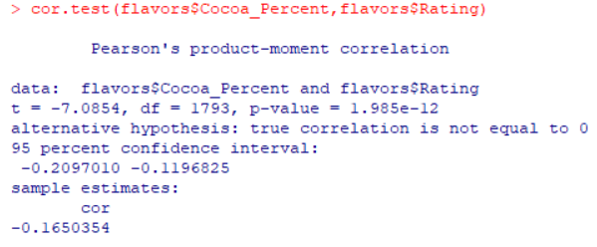The t-statistic is equal to -7.0854, df = 1793, p-value = 1.985*10-12 < 0.05. Thus, at 5% significance level, we reject the null hypothesis and conclude that there is significant correlation between the two variables.

Therefore, we conclude that there is a weak, negative linear relationship between cocoa percentages and ratings assigned to the chocolate bar.

## Exam Question: Use ANOVA to analyze the average ratings assigned to chocolate bars varied by the year of ratings

Exam Solution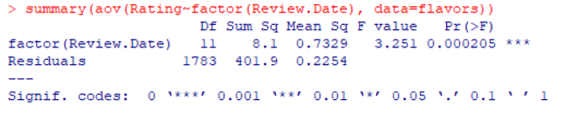The ANOVA results indicate that the F-value is 3.251, p-value = 0.000205 < 0.05. Thus, at a 5% significance level, we reject the null hypothesis and conclude that the average ratings of each review year are not equal.

The following boxplot shows the ratings for each review year. It appears that post 2010, the median ratings were better than years before.## Exam Question: Use T-test analysis to analyze if high cocoa percentage chocolate bars had different ratings than lower cocoa percentage bars

Exam Solution

We will use a t-test to analyze if high cocoa percentage chocolate bars had different ratings than lower cocoa percentage bars. We converted the cocoa percentage variable into a binary variable, High Cocoa, which would store the value of 1 if the cocoa percentage was greater than 70% and 0 otherwise.The t-test was conducted to measure the average ratings of the High Cocoa and Low Cocoa content chocolate bars. The t-statistic is equal to 6.157, df = 1793, p-value = 9.13*10-10 < 0.05. Therefore, at 5% significance level, we reject the null hypothesis and conclude that the average ratings of High Cocoa and Low Cocoa content chocolate bars are different.

Appendix:

R-Code:

library(ggplot2)

library(dplyr)

library(tidyverse)

flavors$Cocoa_Percent<-100*flavors$Cocoa_Percent

str(flavors)

#Descriptives

table(flavors$Company.Location) summary(flavors$Rating)

table(flavors$Rating) ggplot(flavors, aes(x=Rating)) + geom_histogram(color="blue", fill="white", binwidth=0.25)+labs(title="Histogram of Ratings",y="Frequency")+theme_classic() #Origin analysis of favored beans n<-tapply(flavors$Rating,flavors$Broad.Bean.Origin,mean) n[order(-n)] q<-tapply(flavors$Rating,flavors$Bean_Origin,mean) q[order(-q)] #Companies with highest rated bars r<-tapply(flavors$Rating,flavors$Company,mean) r[order(-r)] #Correlation between cocoa percent and ratings ggplot(flavors, aes(x=factor(Rating), y = Cocoa_Percent)) + geom_boxplot(color="blue", fill="white")+labs(title="Boxplot of Ratings and Cocoa Percentages",y="Frequency")+theme_classic() cor(flavors$Cocoa_Percent,flavors$Rating) cor.test(flavors$Cocoa_Percent,flavors$Rating) #ANOVA analysis ggplot(flavors, aes(x=factor(Review.Date), y = Rating)) + geom_boxplot(color="blue", fill="white")+labs(title="Boxplot of Ratings and Year of Ratings",y="Frequency", x="Review Year")+theme_classic() summary(aov(Rating~factor(Review.Date), data=flavors)) #T-test analysis summary(flavors$Cocoa_Percent)

flavors$High_Cocoa<-ifelse(flavors$Cocoa_Percent >70,1,0)

str(flavors)

flavors$High_Cocoa<-as.factor(flavors$High_Cocoa)

t.test(flavors$Rating ~ flavors$High_Cocoa, paired=F, var.equal = T)